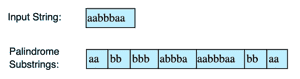# Find All Palindrome Substrings

## Problem Statement

Given a string find all non-single letter substrings that are palindromes. For instance:## Hint

• Find substrings

## Try it yourself

int find_all_palindrome_substrings(string & input) {  //TODO: Write - Your - Code  return -1;}

## Solution

int find_palindromes_in_sub_string(const string& input, int j, int k) {  int count = 0;  for (; j >= 0 && k < input.length(); --j, ++k) {    if (input[j] != input[k]) {            break;    }     cout << input.substr(j, k - j + 1) << endl;    ++count;  }  return count;}int find_all_palindrome_substrings(const string& input) {  int count = 0;  for (int i = 0; i < input.length(); ++i) {        count += find_palindromes_in_sub_string(input, i - 1, i + 1);    count += find_palindromes_in_sub_string(input, i, i + 1);  }  return count;}int main() {  string str = "aabbbaa";  cout << "Total palindrome substrings: "  << find_all_palindrome_substrings(str) << endl;}

## Solution Explanation

### Runtime complexity

The runtime complexity of this solution is polynomial, $O(n^{2})$

### Memory complexity

The memory complexity of this solution is constant, O(1).

### Solution Breakdown

For each letter in the input string, start expanding to the left and right while checking for even and odd length palindromes. Move to the next letter if we know a palindrome doesn’t exist.

We expand one character to the left and right and compare them. If both of them are equal, we print out the palindrome substring.

Practice problems like this and many more by checking out our Grokking the Coding Interview: Patterns for Coding Questions course!

Learn in-demand tech skills in half the time